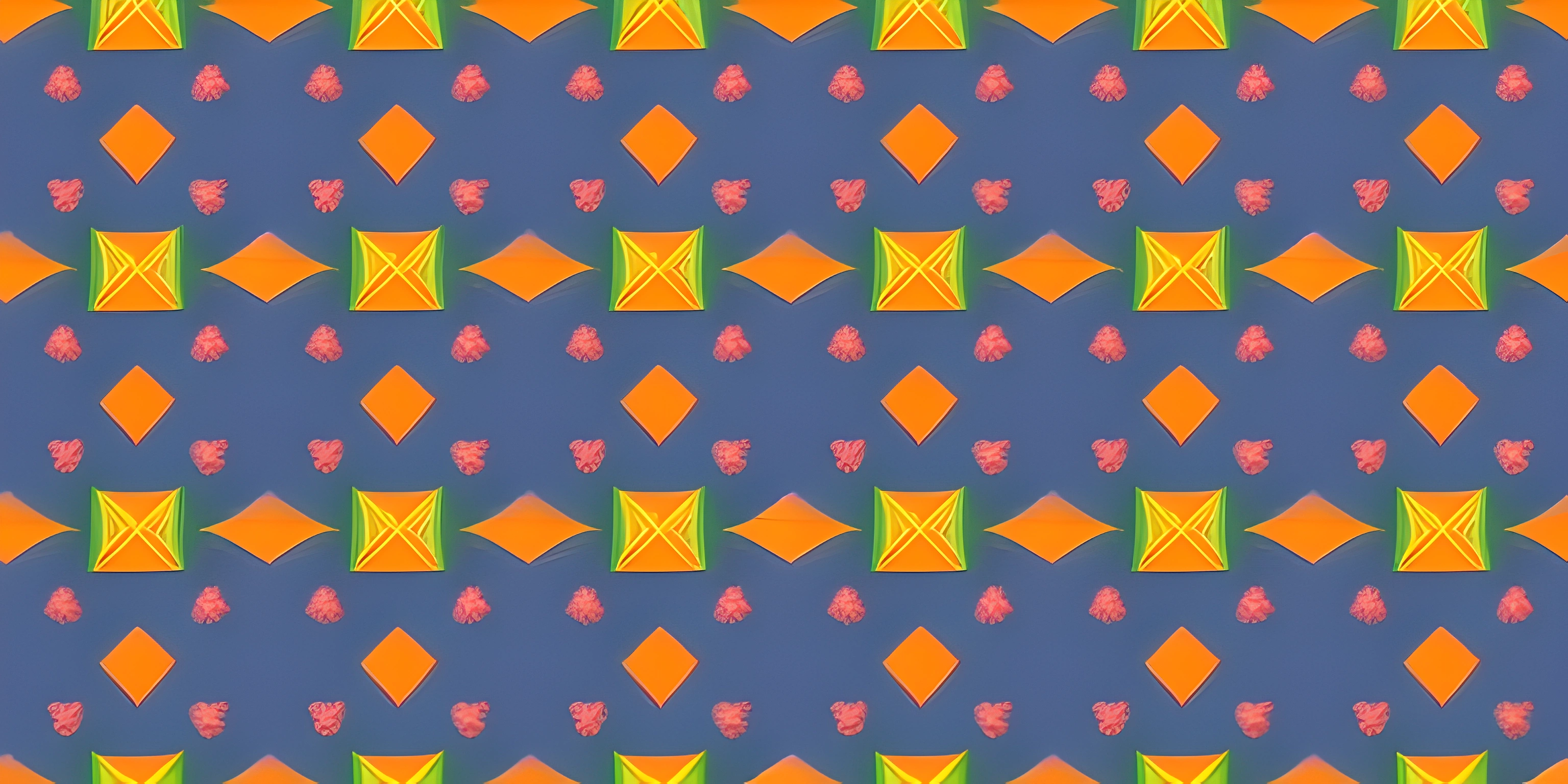# p5 Vector BasicsNote: this page has been created with the use of AI. Please take caution, and note that the content of this page does not necessarily reflect the opinion of Cratecode.

When diving into the world of creative programming, you're bound to come across the concept of vectors. In this article, we'll explore the basics of p5 Vectors, their properties, and how they can be used in various use cases to make your programming more efficient and expressive.

## What is a Vector?

In the context of p5.js, a vector is a mathematical object that has both magnitude and direction. It can be thought of as an arrow pointing from one point to another in a 2D or 3D space. Vectors are incredibly useful in creative programming, as they can be used to represent various properties of objects, such as position, velocity, and acceleration.

## p5 Vector Properties

In p5.js, vectors are represented by the p5.Vector object. This object has several properties, including `x`, `y`, and `z`, which define the components of the vector in 2D or 3D space. You can create a new p5.Vector by using the `createVector()` function:

``let myVector = createVector(1, 2); // Creates a 2D vector with x=1 and y=2``

## Vector Operations

p5.js provides a variety of built-in methods for performing common vector operations. These operations can be used to modify vectors directly or to create new vectors based on existing ones. Some of the most commonly used vector operations are:

• `add()`: Adds two vectors together, component-wise.
• `sub()`: Subtracts one vector from another, component-wise.
• `mult()`: Multiplies a vector by a scalar (a single number).
• `div()`: Divides a vector by a scalar.
• `mag()`: Calculates the magnitude (length) of a vector.
• `normalize()`: Scales a vector to have a magnitude of 1 (unit vector).

Here's a simple example using these operations:

``````let v1 = createVector(1, 2);
let v2 = createVector(3, 4);

let v3 = v1.add(v2); // Adds the two vectors together -> (4, 6)
v3.mult(2); // Multiplies the vector by a scalar -> (8, 12)

let magnitude = v3.mag(); // Calculates the magnitude -> 14.1421...
v3.normalize(); // Scales the vector to have a magnitude of 1 -> (0.566, 0.849)``````

## Use Cases for p5 Vectors

Vectors can be used in a wide variety of creative programming scenarios, such as:

• Position: Representing the position of an object in 2D or 3D space.
• Velocity: Representing the speed and direction of an object's movement.
• Acceleration: Representing the rate of change of an object's velocity.
• Forces: Modeling the effects of forces, such as gravity or friction, on objects.

By using vectors to represent these properties, you can create complex and expressive simulations with ease. For example, you could model the motion of a bouncing ball by using vectors for its position, velocity, and acceleration, and then update these vectors based on the laws of physics.

``````let position = createVector(0, 0);
let velocity = createVector(1, -2);
let acceleration = createVector(0, 0.1);

function update() {